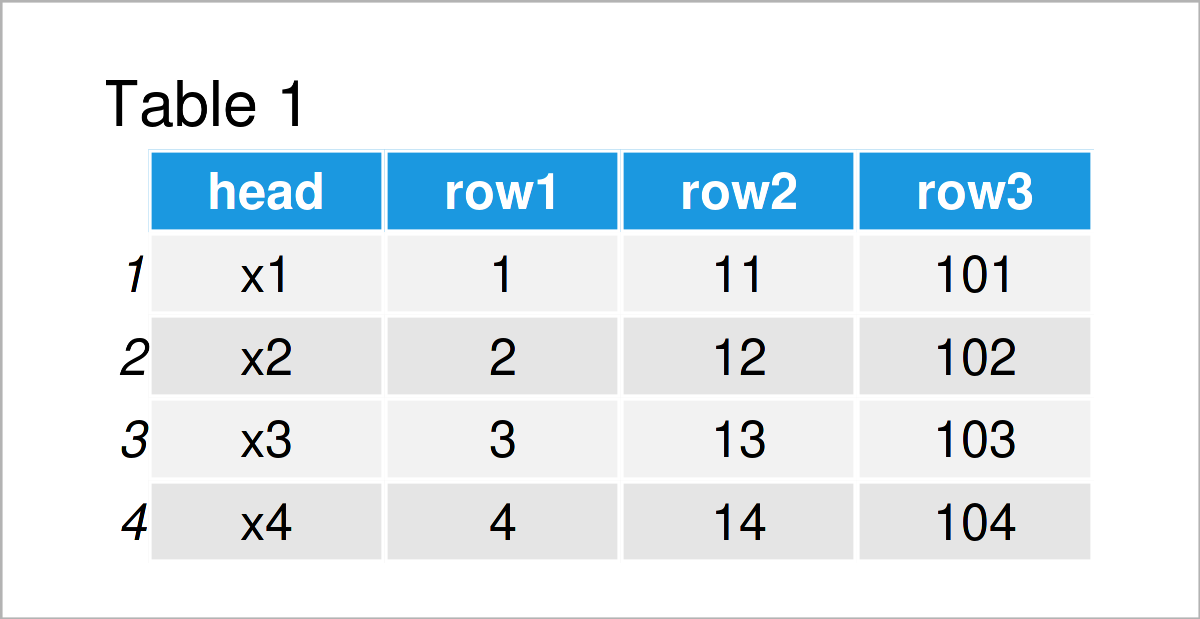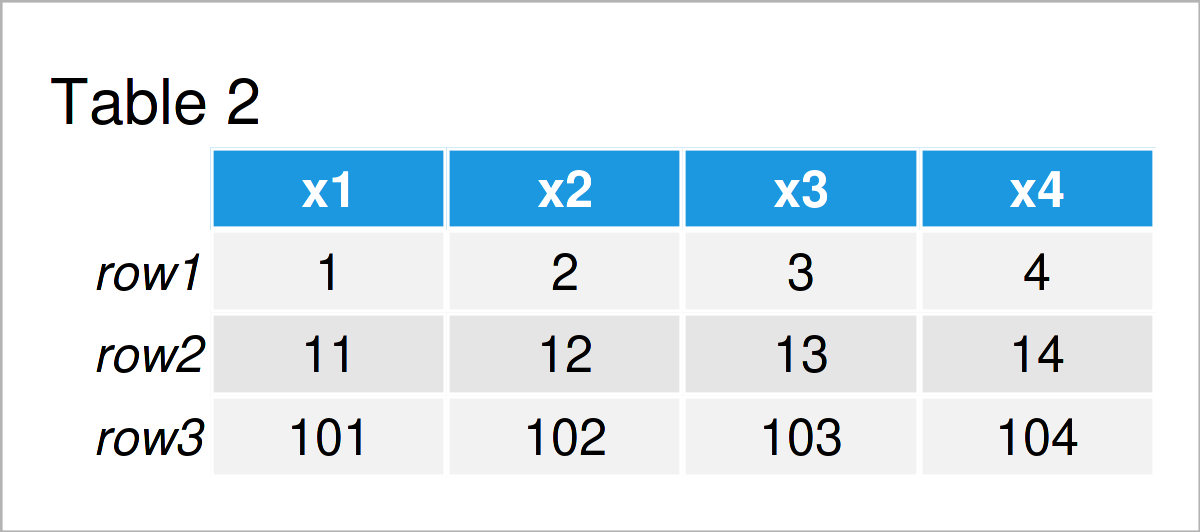# Transpose Data Frame & Set First Column as Header in R (Example)

In this tutorial, I’ll illustrate how to transpose a data matrix and use the first variable as column names in R.

The article contains one example for the transposing of a data matrix and with the first variable as header. To be more specific, the article will contain these topics:

Let’s dive right in…

## Creating Example Data

The following data is used as basement for this R programming language tutorial:

```data <- data.frame(head = paste0("x", 1:4),                  # Create example data
row1 = 1:4,
row2 = 11:14,
row3 = 101:104)
data                                                         # Print example data```Table 1 shows that our example data consists of four rows and four columns called “head”, “row1”, “row2”, and “row3”.

## Example: Transposing Data Frame & Maintaining First Column as Heading

This section demonstrates how to transpose a data matrix and use the first column of the input data frame as header.

For this task, we can use the t, data.frame, and setNames functions as shown below:

```data_t <- setNames(data.frame(t(data[ , - 1])), data[ , 1])  # Transpose data
data_t                                                       # Print transposed data```As shown in Table 2, the previous R code has created a transposed version of our data with the first variable as column names.

## Video, Further Resources & Summary

I have recently released a video on my YouTube channel, which explains the examples of this article. You can find the video below.

Besides the video, you may read the related R programming tutorials on my homepage. I have released several articles that are related to the transposing of a data matrix and with the first variable as header already.

In summary: In this R tutorial you have learned how to rotate a data frame and keep the first variable as column names. In case you have additional questions, please let me know in the comments.

Subscribe to the Statistics Globe Newsletter